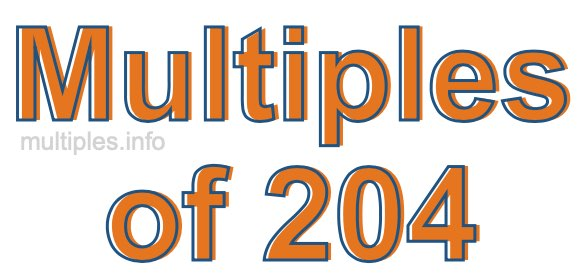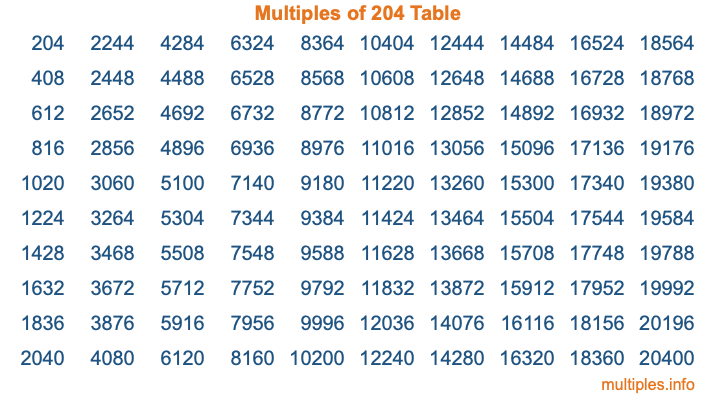Multiples of 204Welcome to the Multiples of 204 page. Here we will first teach you everything you will ever need to know about the multiples of 204, and then give you a study guide summary of everything we taught you to make sure you remember it all. Use this page to look up facts and learn information about the multiples of 204. This page will make you a multiples of two hundred four expert!

Definition of Multiples of 204
Multiples of 204 are all the numbers that when divided by 204 equal an integer. Each of the multiples of 204 are called a multiple. A multiple of 204 is created by multiplying 204 by an integer.

Therefore, to create a list of multiples of 204, you start with 1 multiplied by 204, then 2 multiplied by 204, then 3 multiplied by 204, and so on for as long as you want. Thus, the list of the first five multiples of 204 is 204, 408, 612, 816, and 1020. To see a larger list of multiples of 204, see the printable image of Multiples of 204 further down on this page. We also have a category where you can choose any nth multiple of 204.

Multiples of 204 Checker
The Multiples of 204 Checker below checks to see if any number of your choice is a multiple of 204. In other words, it checks to see if there is any number (integer) that when multiplied by 204 will equal your number. To do that, we divide your number by 204. If the the quotient is an integer, then your number is a multiple of 204.

Is  a multiple of 204?

Least Common Multiple of 204 and ...
A Least Common Multiple (LCM) is the lowest multiple that two or more numbers have in common. This is also called the smallest common multiple or lowest common multiple and is useful to know when you are adding our subtracting fractions. Enter one or more numbers below (204 is already entered) to find the LCM.

Check out our LCM Calculator if you need more details about the Least Common Multiple or if you need the LCM for different numbers for adding and subtraction fractions.

nth Multiple of 204
As we stated above, 204 is the first multiple of 204, 408 is the second multiple of 204, 612 is the third multiple of 204, and so on. Enter a number below to find the nth multiple of 204.

th multiple of 204

Multiples of 204 vs Factors of 204
204 is a multiple of 204 and a factor of 204, but that is where the similarities end. All postive multiples of 204 are 204 or greater than 204. All positive factors of 204 are 204 or less than 204.

Below is the beginning list of multiples of 204 and the factors of 204 so you can compare:

Multiples of 204: 204, 408, 612, 816, 1020, etc.

Factors of 204: 1, 2, 3, 4, 6, 12, 17, 34, 51, 68, 102, 204

As you can see, the multiples of 204 are all the numbers that you can divide by 204 to get a whole number. The factors of 204, on the other hand, are all the whole numbers that you can multiply by another whole number to get 204.

It's also interesting to note that if a number (x) is a factor of 204, then 204 will also be a multiple of that number (x).

Multiples of 204 vs Divisors of 204
The divisors of 204 are all the integers that 204 can be divided by evenly. Below is a list of the divisors of 204.

Divisors of 204: 1, 2, 3, 4, 6, 12, 17, 34, 51, 68, 102, 204

The interesting thing to note here is that if you take any multiple of 204 and divide it by a divisor of 204, you will see that the quotient is an integer.

Multiples of 204 Table
Below is an image of the first 100 multiples of 204 in a table. The table is in chronological order, column by column. The first column has the first ten multiples of 204, the second column has the next ten multiples of 204, and so on.The Multiples of 204 Table is also referred to as the 204 Times Table or Times Table of 204. You are welcome to print out our table for your studies.

Negative Multiples of 204
Although not often discussed or needed in math, it is worth mentioning that you can make a list of negative multiples of 204 by multiplying 204 by -1, then by -2, then by -3, and so on, to get the following list of negative multiples of 204:

-204, -408, -612, -816, -1020, etc.

Multiples of 204 Summary
Below is a summary of important Multiples of 204 facts that we have discussed on this page. To retain the knowledge on this page, we recommend that you read through the summary and explain to yourself or a study partner why they hold true.

There are an infinite number of multiples of 204.

A multiple of 204 divided by 204 will equal a whole number.

204 divided by a factor of 204 equals a divisor of 204.

The nth multiple of 204 is n times 204.

The largest factor of 204 is equal to the first positive multiple of 204.

204 is a multiple of every factor of 204.

204 is a multiple of 204.

A multiple of 204 divided by a divisor of 204 equals an integer.

204 divided by a divisor of 204 equals a factor of 204.

Any integer times 204 will equal a multiple of 204.

Multiples of a Number
Here you can get the multiples of another number, all with the same attention to detail as we did for multiples of 204 on this page.

Multiples of
Multiples of 205
Did you find our page about multiples of two hundred four educational? Do you want more knowledge? Check out the multiples of the next number on our list!